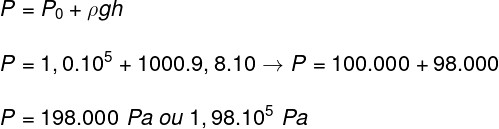Mechanics

# Fluids

Fluids are substances capable of flowing and that are easily deformed. They include liquids, gases and even plasma. Ideal fluids do not offer any resistance to the application of forces, to the point of assuming the same shape as the container in which they are confined.

## Fluid characteristics

Fluids can be divided initially into two categories: ideal fluids and real fluids. In this article we will deal with ideal fluids . To do so, let’s check out some of its main features :

• they do not have viscosity;
• do not resist cuts;
• they are incompressible;
• do not show turbulence.

In addition to these characteristics, since fluids do not have a defined shape, their properties are studied based on quantities such as specific mass and pressure that they are capable of exerting.

## Especific mass

The specific mass of a fluid refers to the amount of matter contained in a certain volume . It can be calculated as the mass of the fluid divided by the volume occupied by it.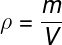ρ – specific mass

m – mass

V – volume

The unit of measurement of specific mass, according to the I nternational System of Units , is kg/m³ , however it is common to use units such as og/cm³ or even kg/L.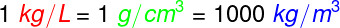The specific mass of fluids is defined based on the density of water, which has a density of 1000 kg/m³, 1 g/cm³ or 1 kg/L. In the following table, we will check the density of other known fluids.

 Substance Specific mass (g/cm³) Alcohol 0.79 Benzene 0.90 Water 1.00 Mercury 13.60 hexane 0.66 nitroglycerin 1.60

hydrostatic pressure

Fluids at rest are capable of exerting pressure, which is proportional to their density and height . Hydrostatic pressure , also known as gauge pressure , can be calculated as the product of specific mass, gravity acceleration , and fluid height.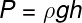ρ – specific mass (kg/m³)

g – acceleration due to gravity (m/s²)

h – height of the fluid in relation to the surface (m)

From Stevin ‘s theorem , it is also possible to determine what the pressure is at any point in the interior of a fluid. Therefore, it must be taken into account whether or not the fluid is subjected to an external pressure , such as atmospheric pressure.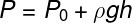0 – atmospheric pressure

We call atmospheric pressure the pressure exerted by the fluid that makes up the earth’s atmosphere. At sea level, the Earth’s atmospheric pressure is approximately 1.01 x 10 5 Pa (pascal), and 1 Pa is equivalent to a force of 1 N applied over an area of ​​1 m².

## Pascal’s Theorem

According to Pascal’s Theorem, all pressure exerted on a fluid is uniformly distributed within it. Furthermore, the force that the fluid exerts on the container that confines it is always perpendicular to the surface of the container. Want to understand better about it? Access our specific article: Pascal ‘s Theorem .

## Archimedes’ theorem

According to Archimedes’ theorem, the volume of fluid that is displaced when we insert a body into a fluid corresponds to the volume of the body inserted. Also, if the weight of the volume of fluid displaced is less than the weight of the body placed on top of the fluid, then that body will sink. If you want to know more about the subject, access our specific article: Theorem of Archimedes .

## fluid dynamics

Fluid dynamics is the field of mechanics that studies fluids in motion. Let us illustrate the situation where an ideal fluid flows through a tube of different cross-sectional areas and different heights.

The main equation of fluid dynamics is the Bernoulli equation . Through it, it is possible to relate different points of the fluid illustrated in the previous image.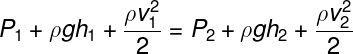1 and P 2 – pressures at points 1 and 2

1 and h 2 – heights of points 1 and 2

1 and v 2 – fluid flow velocities at points 1 and 2

Bernoulli’s equation is derived from the conservation of mechanical energy . For it to be valid, however, it is necessary that the studied fluid is ideal. This equation has several practical applications, such as aerodynamics and hydrodynamics.

The flight of airplanes , for example, is explained through the Bernoulli equation . Air flowing over the top of an airplane’s wing flows faster than air flowing over the underside of the wing. This causes the pressure on top of the wing to be less than the pressure on the underside, thus creating a lift force capable of keeping the plane in the air.

## Solved exercises on fluids

Question 1 — An ideal fluid occupies a volume of 0.5 cm³ and has a mass of 0.2 g. Determine the density of this fluid in g/cm³ and choose the correct alternative.

a) 0.8

b) 0.4

c) 1.2

d) 0.9

Resolution:

To solve the exercise, we just need to calculate the specific mass by dividing the mass of the fluid by the volume it occupies. Watch: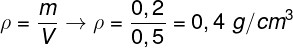Question 2 — An ideal fluid of specific mass equal to 0.8 g/cm³ is in hydrostatic equilibrium when stored in a rigid and closed container, thus occupying its entire volume. Knowing that the area of ​​the base of the cylindrical container is equal to 0.25 m² and its height is 1.25 m, the hydrostatic pressure exerted by the fluid is equal to:

Data: g = 10 m/s².

a) 125 Pa

b) 50 Pa

c) 10 Pa

d) 15 Pa

Resolution:

Note that the pressure exerted by the fluid does not depend on the area of ​​the base of the container, but only on its depth. To calculate this pressure, we simply use the formula for hydrostatic pressure, also known as gauge pressure. Watch: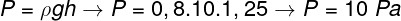Question 3 — A diver is at a depth of 10 m in a lake whose water has a density of 1000 kg/m³. Knowing that the gravity acceleration at the location is approximately 9.8 m/s², the total pressure exerted on the diver is:

Data: P 0 = 1.0.10 5 Pa.

a) 1.98.10 5 Pa

b) 2.98.10 5 Pa

c) 0.98.10 5 Pa

d) 1.89.10 4 Pa

Resolution:

To calculate the pressure exerted on the diver, it is necessary to consider the atmospheric pressure, in addition to the pressure exerted by the water. For this, we use Stevin’s theorem: